• Python画图示例（1） 一维数据集绘图 Python画图示例（2） 二维数据集绘图 Python画图示例（3） 其他绘图样式,散点图,直方图等 Python画图示例（4） 3D绘图 目录 1、用 Numpy ndarray 作为数据传入 ply 2....
Python画图示例（1） 一维数据集绘图
Python画图示例（2） 二维数据集绘图
Python画图示例（3） 其他绘图样式,散点图,直方图等
Python画图示例（4） 3D绘图
目录

1、用 Numpy ndarray 作为数据传入 ply
2.操纵坐标轴和增加网格及标签的函数
3.plt.xlim 和 plt.ylim 设置每个坐标轴的最小值和最大值
4. 添加标题和标签 plt.title, plt.xlabe, plt.ylabel 离散点, 线

1、用 Numpy ndarray 作为数据传入 ply
import numpy as np
import matplotlib as mpl
import matplotlib.pyplot as plt

np.random.seed(1000)
y = np.random.standard_normal(10)
x = range(len(y))

plt.plot(y)
plt.show()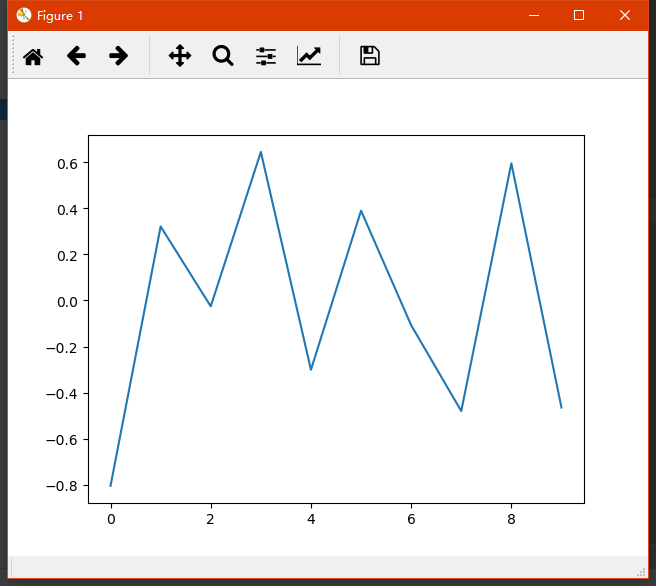2.操纵坐标轴和增加网格及标签的函数
import numpy as np
import matplotlib as mpl
import matplotlib.pyplot as plt

np.random.seed(1000)
y = np.random.standard_normal(10)
plt.plot(y.cumsum())
plt.grid(True) ##增加格点
plt.axis('tight') # 坐标轴适应数据量 axis 设置坐标轴
plt.show()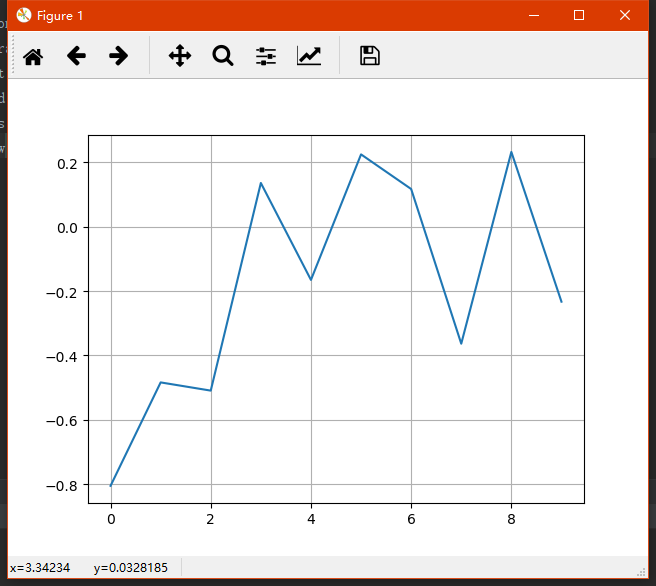3.plt.xlim 和 plt.ylim 设置每个坐标轴的最小值和最大值
import numpy as np
import matplotlib as mpl
import matplotlib.pyplot as plt

np.random.seed(1000)
y = np.random.standard_normal(20)
plt.plot(y.cumsum())
plt.grid(True) ##增加格点
plt.xlim(-1,20)
plt.ylim(np.min(y.cumsum())- 1, np.max(y.cumsum()) + 1)

plt.show()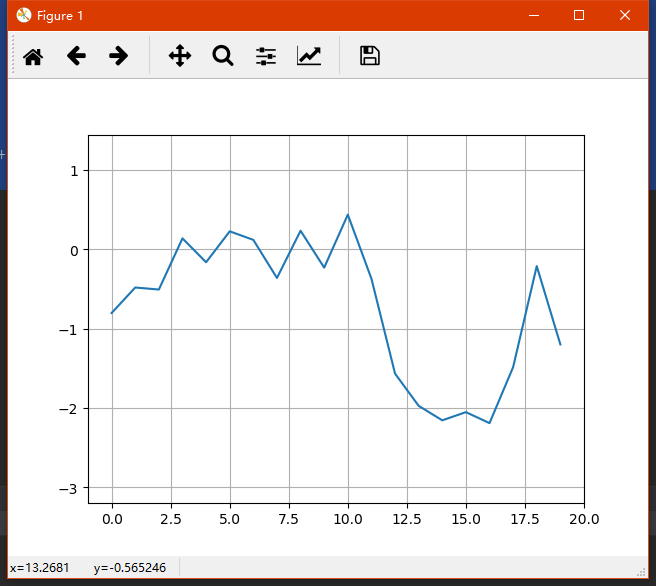4. 添加标题和标签 plt.title, plt.xlabe, plt.ylabel 离散点, 线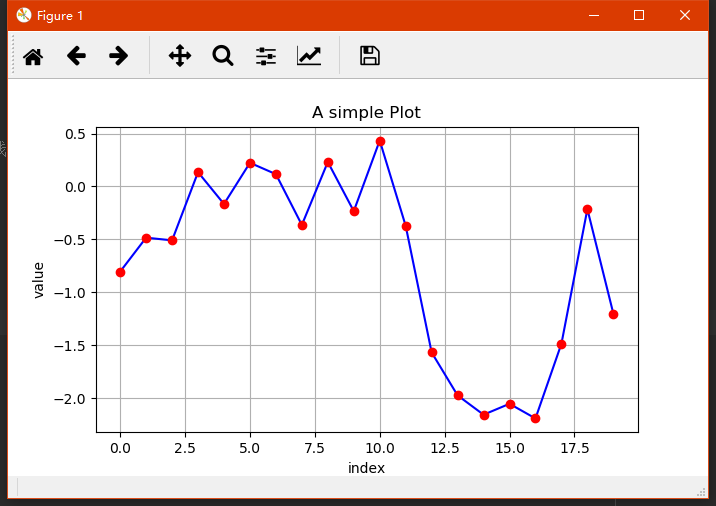展开全文python
• KL散度 KL 散度是种衡量两个概率分布的匹配程度的指标，两个分布...第组：数据集为采集100个人的年龄，为以下表格所示，我们使用KL散度去研究最符合的分布类型。 age 0 1 2 3 4 5 6 ...
KL散度
KL 散度是一种衡量两个概率分布的匹配程度的指标，两个分布差异越大，KL散度越大。
定义：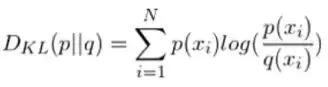其中 p(x) 是真实分布，q(x)是目标分布（建模目标分布），如果两个分布完全匹配，那么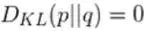第一组：数据集为采集100个人的年龄，为以下表格所示，我们使用KL散度去研究最符合的分布类型。
age012345678910总数count3671113181511754100
尝试1：使用均匀分布建立模型
可视化为：黄色的为建立的目标均匀分布模型，与蓝色真实分布的对比。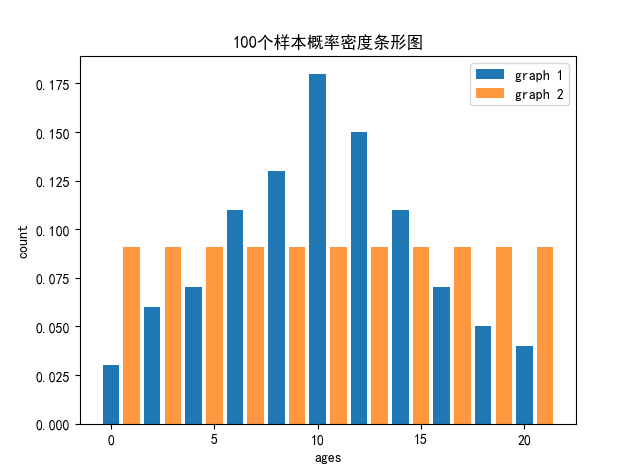尝试2：使用高斯分布建立模型
可视化为：（红色虚线是相同与拟合的一条正态分布曲线，蓝色条形图为概率密度）：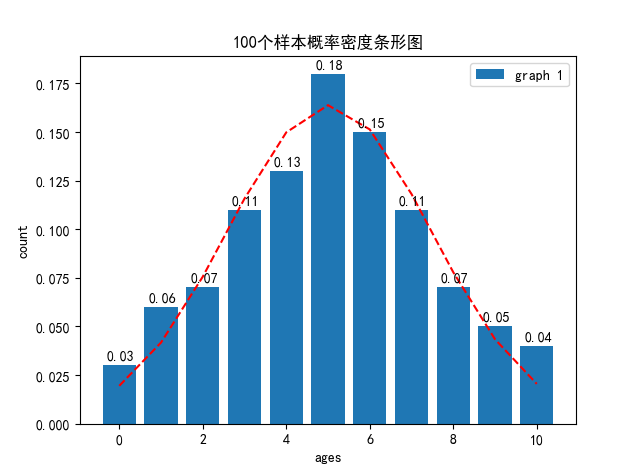计算分析 ：
如何判断真实分布更接近均匀分布还是高斯分布，用肉眼看是很不准确的，用KL散度来衡量真实分布去匹配目标分布所损失的信息量。所以能够将模型量化去比较从而判断出接近哪种分布。
1、计算与均匀分布的KL散度：
import numpy as np
import math
count=np.array([3,6,7,11,13,18,15,11,7,5,4])
count_rate=count/100
balance_rate=1/11
sum=0
for i in range(11):
sum+=count_rate[i]*math.log(count_rate[i]/balance_rate)
print(sum)
计算结果为：0.12899493763053263

2、计算与高斯分布的KL散度：

def gaosi(x):
mu=5.03
sigma=2.4349743325135895
t1=1/(sigma*math.sqrt(2*math.pi))
t2=((x-mu)**2)/(2*sigma*sigma)
return math.exp(-1*t2)*t1

count=np.array([3,6,7,11,13,18,15,11,7,5,4])
count_rate=count/100

sum=0
for i in range(11):
sum+=count_rate[i]*math.log(count_rate[i]/gaosi(i))
print(sum)
计算结果为：0.03997441345364968

结论：
在只考虑均匀分布模型与高斯分布模型的情况下，用本身去拟合目标模型时，匹配高斯分布所损失的信息量最少，计算发现该数据集的分布更符合高斯分布。
展开全文KL散度 高斯分布
• Python画图示例（1） 一维数据集绘图 Python画图示例（2） 二维数据集绘图 Python画图示例（3） 其他绘图样式,散点图,直方图等 Python画图示例（4） 3D绘图 目录 1.两个数据集绘图 2.添加图例 plt.legend(loc ...
Python画图示例（1） 一维数据集绘图
Python画图示例（2） 二维数据集绘图
Python画图示例（3） 其他绘图样式,散点图,直方图等
Python画图示例（4） 3D绘图
目录
1.两个数据集绘图
2.添加图例 plt.legend(loc = 0)
3.使用2个 Y轴(左右)fig, ax1 = plt.subplots() ax2 = ax1.twinx()
4.使用两个子图(上下,左右)plt.subplot(211)
5.左右子图

1.两个数据集绘图
import numpy as np
import matplotlib as mpl
import matplotlib.pyplot as plt

np.random.seed(2000)
y = np.random.standard_normal((10, 2))
plt.figure(figsize=(7,5))
plt.plot(y, lw = 1.5)
plt.plot(y, 'ro')
plt.grid(True)
plt.axis('tight')
plt.xlabel('index')
plt.ylabel('value')
plt.title('A simple plot')
plt.show()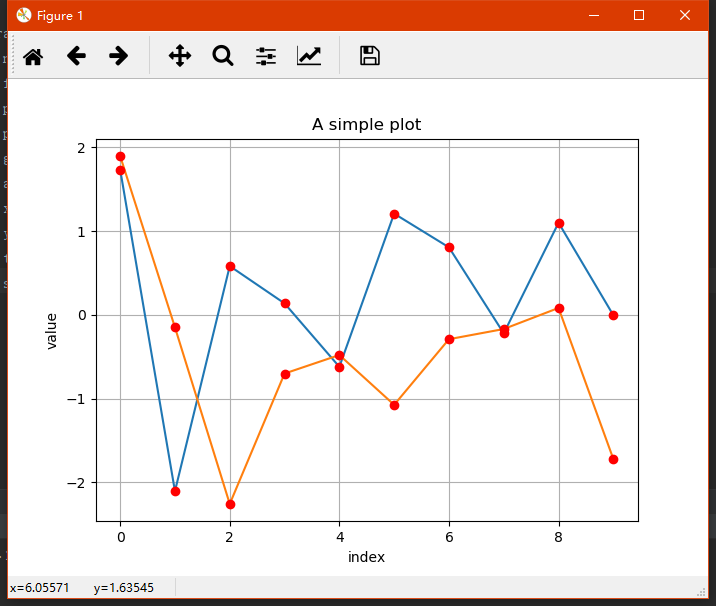2.添加图例 plt.legend(loc = 0)
import numpy as np
import matplotlib as mpl
import matplotlib.pyplot as plt

np.random.seed(2000)
y = np.random.standard_normal((10, 2))
plt.figure(figsize=(7,5))
plt.plot(y[:,0], lw = 1.5,label = '1st')
plt.plot(y[:,1], lw = 1.5, label = '2st')
plt.plot(y, 'ro')
plt.grid(True)
plt.legend(loc = 0) #图例位置自动
plt.axis('tight')
plt.xlabel('index')
plt.ylabel('value')
plt.title('A simple plot')
plt.show()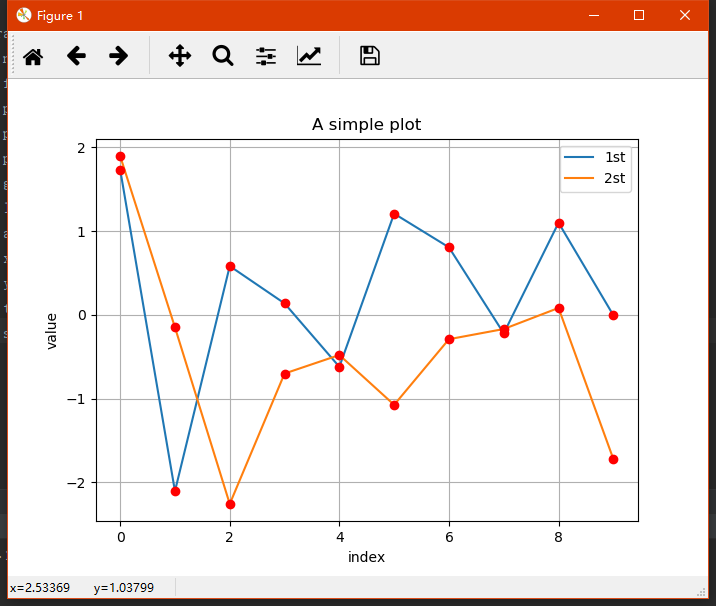3.使用2个 Y轴(左右)fig, ax1 = plt.subplots() ax2 = ax1.twinx()
import numpy as np
import matplotlib as mpl
import matplotlib.pyplot as plt

np.random.seed(2000)
y = np.random.standard_normal((10, 2))

fig, ax1 = plt.subplots() # 关键代码1 plt first data set using first (left) axis

plt.plot(y[:,0], lw = 1.5,label = '1st')

plt.plot(y[:,0], 'ro')
plt.grid(True)
plt.legend(loc = 0) #图例位置自动
plt.axis('tight')
plt.xlabel('index')
plt.ylabel('value')
plt.title('A simple plot')

ax2 = ax1.twinx()  #关键代码2  plt second data set using second(right) axis
plt.plot(y[:,1],'g', lw = 1.5, label = '2nd')
plt.plot(y[:,1], 'ro')
plt.legend(loc = 0)
plt.ylabel('value 2nd')
plt.show()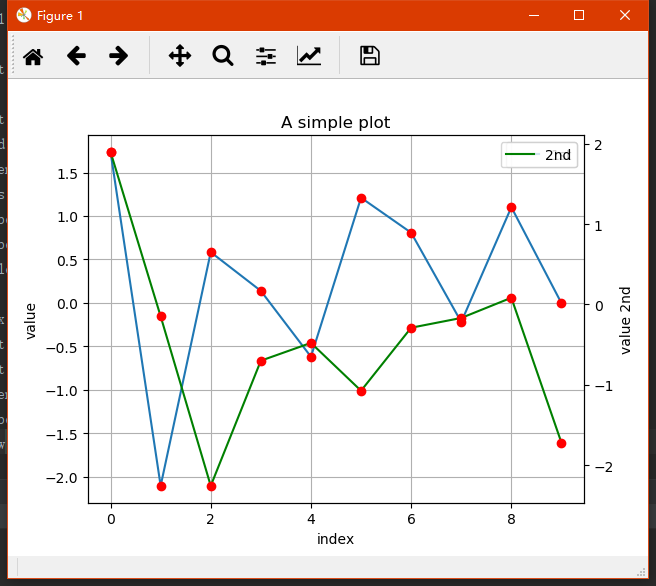4.使用两个子图(上下,左右)plt.subplot(211)
通过使用 plt.subplots 函数,可以直接访问底层绘图对象,例如可以用它生成和第一个子图共享 x 轴的第二个子图.
import numpy as np
import matplotlib as mpl
import matplotlib.pyplot as plt

np.random.seed(2000)
y = np.random.standard_normal((10, 2))

plt.figure(figsize=(7,5))
plt.subplot(211)  #两行一列,第一个图
plt.plot(y[:,0], lw = 1.5,label = '1st')
plt.plot(y[:,0], 'ro')
plt.grid(True)
plt.legend(loc = 0) #图例位置自动
plt.axis('tight')
plt.ylabel('value')
plt.title('A simple plot')

plt.subplot(212) #两行一列.第二个图
plt.plot(y[:,1],'g', lw = 1.5, label = '2nd')
plt.plot(y[:,1], 'ro')
plt.grid(True)
plt.legend(loc = 0)
plt.xlabel('index')
plt.ylabel('value 2nd')
plt.axis('tight')
plt.show()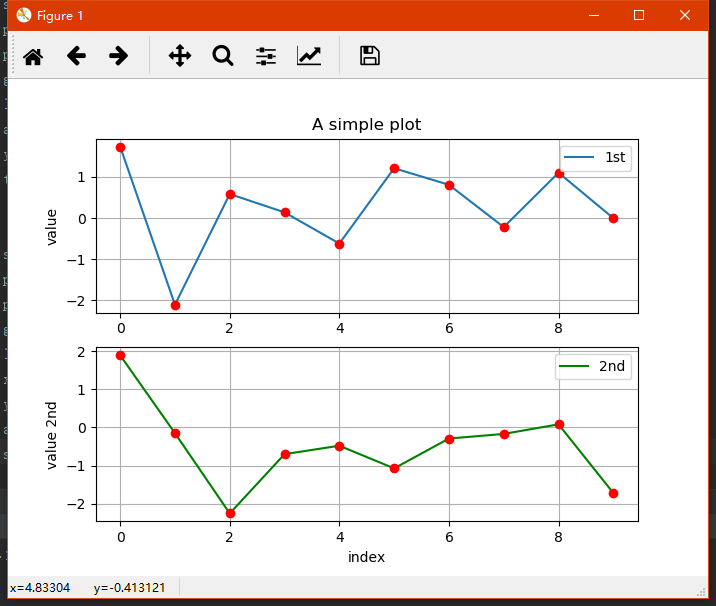5.左右子图
有时候,选择两个不同的图标类型来可视化数据可能是必要的或者是理想的.利用子图方法:
import numpy as np
import matplotlib as mpl
import matplotlib.pyplot as plt

np.random.seed(2000)
y = np.random.standard_normal((10, 2))

plt.figure(figsize=(10,5))
plt.subplot(121)  #两行一列,第一个图
plt.plot(y[:,0], lw = 1.5,label = '1st')
plt.plot(y[:,0], 'ro')
plt.grid(True)
plt.legend(loc = 0) #图例位置自动
plt.axis('tight')
plt.xlabel('index')
plt.ylabel('value')
plt.title('1st Data Set')

plt.subplot(122)
plt.bar(np.arange(len(y)), y[:,1],width=0.5, color='g',label = '2nc')
plt.grid(True)
plt.legend(loc=0)
plt.axis('tight')
plt.xlabel('index')
plt.title('2nd Data Set')
plt.show()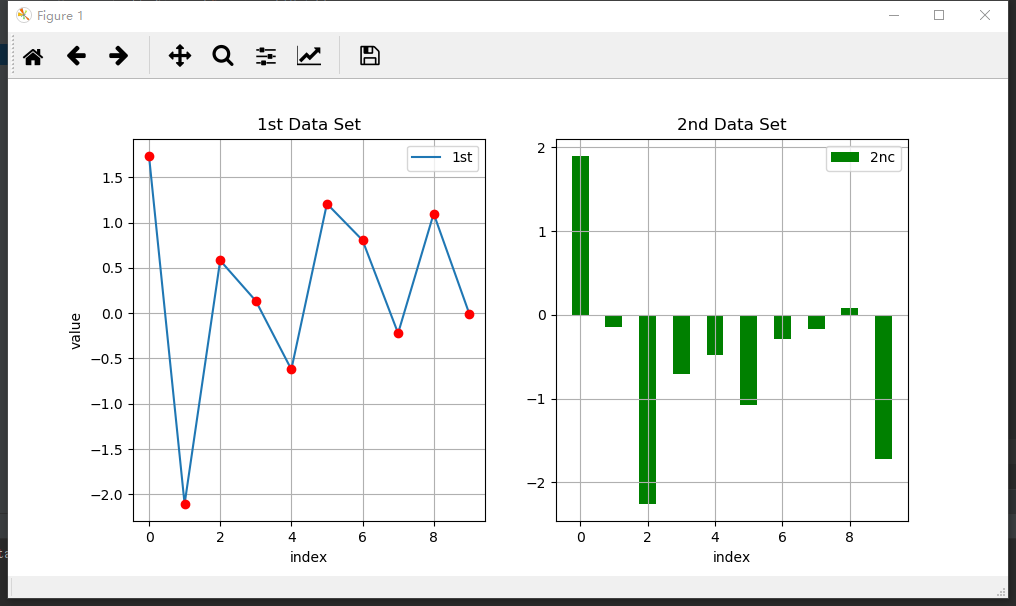展开全文python
• 神经网络对于一维数据非常重要，时序数据集、信号处理数据集和一些文本嵌入数据集都是一维数据，会频繁的使用到神经网络。我们在此利用一组一维数据构造卷积层-最大池化层-全连接层的卷积神经网络。希望给大家使用...
使用一维数据构造简单卷积神经网络
觉得有用的话,欢迎一起讨论相互学习~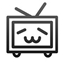神经网络对于一维数据非常重要，时序数据集、信号处理数据集和一些文本嵌入数据集都是一维数据，会频繁的使用到神经网络。我们在此利用一组一维数据构造卷积层-最大池化层-全连接层的卷积神经网络。希望给大家使用CNN处理一维数据一些帮助。
参考代码
# Implementing Different Layers
# ---------------------------------------
#
# We will illustrate how to use different types
# of layers in TensorFlow
#
# The layers of interest are:
#  (1) Convolutional Layer卷积层
#  (2) Activation Layer激活层
#  (3) Max-Pool Layer池化层
#  (4) Fully Connected Layer 全连接层
#
# We will generate two different data sets for this
#  script, a 1-D data set (row of data) and
#  a 2-D data set (similar to picture)

import tensorflow as tf
import matplotlib.pyplot as plt
import csv
import os
import random
import numpy as np
import random
from tensorflow.python.framework import ops

ops.reset_default_graph()

# ---------------------------------------------------|
# -------------------1D-data-------------------------|
# ---------------------------------------------------|

# Create graph session 创建初始图结构
ops.reset_default_graph()
sess = tf.Session()

# parameters for the run运行参数
data_size = 25
conv_size = 5  # 卷积核宽度方向的大小
maxpool_size = 5  # 池化层核宽度方向上的大小
stride_size = 1  # 卷积核宽度方向上的步长

# ensure reproducibility 确保复现性
seed = 13
np.random.seed(seed)
tf.set_random_seed(seed)

# Generate 1D data 生成一维数据
data_1d = np.random.normal(size=data_size)

# Placeholder
x_input_1d = tf.placeholder(dtype=tf.float32, shape=[data_size])

# --------Convolution--------
def conv_layer_1d(input_1d, my_filter, stride):
# TensorFlow's 'conv2d()' function only works with 4D arrays:
# [batch, height, width, channels], we have 1 batch, and
# width = 1, but height = the length of the input, and 1 channel.
# So next we create the 4D array by inserting dimension 1's.
# 关于数据维度的处理十分关键，因为tensorflow中卷积操作只支持四维的张量，
# 所以要人为的把数据补充为4维数据[1,1,25,1]
input_2d = tf.expand_dims(input_1d, 0)
input_3d = tf.expand_dims(input_2d, 0)
input_4d = tf.expand_dims(input_3d, 3)
# Perform convolution with stride = 1, if we wanted to increase the stride,
# to say '2', then strides=[1,1,2,1]
convolution_output = tf.nn.conv2d(input_4d, filter=my_filter, strides=[1, 1, stride, 1], padding="VALID")
# Get rid of extra dimensions 去掉多余的层数，只保留数字
conv_output_1d = tf.squeeze(convolution_output)
return (conv_output_1d)

# Create filter for convolution.
my_filter = tf.Variable(tf.random_normal(shape=[1, conv_size, 1, 1]))
# Create convolution layer
my_convolution_output = conv_layer_1d(x_input_1d, my_filter, stride=stride_size)

# --------Activation--------
def activation(input_1d):
return (tf.nn.relu(input_1d))

# Create activation layer
my_activation_output = activation(my_convolution_output)

# --------Max Pool--------
def max_pool(input_1d, width, stride):
# Just like 'conv2d()' above, max_pool() works with 4D arrays.
# [batch_size=1, width=1, height=num_input, channels=1]
# 因为在处理卷积层的结果时，使用squeeze函数对结果输出进行降维，所以此处要将最大池化层的维度提升为4维
input_2d = tf.expand_dims(input_1d, 0)
input_3d = tf.expand_dims(input_2d, 0)
input_4d = tf.expand_dims(input_3d, 3)
# Perform the max pooling with strides = [1,1,1,1]
# If we wanted to increase the stride on our data dimension, say by
# a factor of '2', we put strides = [1, 1, 2, 1]
# We will also need to specify the width of the max-window ('width')
pool_output = tf.nn.max_pool(input_4d, ksize=[1, 1, width, 1],
strides=[1, 1, stride, 1],
# Get rid of extra dimensions
pool_output_1d = tf.squeeze(pool_output)
return (pool_output_1d)

my_maxpool_output = max_pool(my_activation_output, width=maxpool_size, stride=stride_size)

# --------Fully Connected--------
def fully_connected(input_layer, num_outputs):
# First we find the needed shape of the multiplication weight matrix:
# The dimension will be (length of input) by (num_outputs)
weight_shape = tf.squeeze(tf.stack([tf.shape(input_layer), [num_outputs]]))
# squeeze函数用于去掉维度为1的维度。保留数据。

# Initialize such weight
# 初始化weight
weight = tf.random_normal(weight_shape, stddev=0.1)
# Initialize the bias
# 初始化bias
bias = tf.random_normal(shape=[num_outputs])
# Make the 1D input array into a 2D array for matrix multiplication
# 将一维的数组添加一维成为2维数组
input_layer_2d = tf.expand_dims(input_layer, 0)
# Perform the matrix multiplication and add the bias
# Get rid of extra dimensions
# 去掉多余的维度只保留数据
full_output_1d = tf.squeeze(full_output)
return (full_output_1d)

my_full_output = fully_connected(my_maxpool_output, 5)

# Run graph
# Initialize Variables
init = tf.global_variables_initializer()
sess.run(init)

feed_dict = {x_input_1d: data_1d}

print('>>>> 1D Data <<<<')

# Convolution Output
print('Input = array of length %d'%(x_input_1d.shape.as_list()))  # 25
print('Convolution w/ filter, length = %d, stride size = %d, results in an array of length %d:'%
(conv_size, stride_size, my_convolution_output.shape.as_list()))  # 21
print(sess.run(my_convolution_output, feed_dict=feed_dict))

# Activation Output
print('\nInput = above array of length %d'%(my_convolution_output.shape.as_list()))  # 21
print('ReLU element wise returns an array of length %d:'%(my_activation_output.shape.as_list()))  # 21
print(sess.run(my_activation_output, feed_dict=feed_dict))

# Max Pool Output
print('\nInput = above array of length %d'%(my_activation_output.shape.as_list()))  # 21
print('MaxPool, window length = %d, stride size = %d, results in the array of length %d'%
(maxpool_size, stride_size, my_maxpool_output.shape.as_list()))  # 17
print(sess.run(my_maxpool_output, feed_dict=feed_dict))

# Fully Connected Output
print('\nInput = above array of length %d'%(my_maxpool_output.shape.as_list()))  # 17
print('Fully connected layer on all 4 rows with %d outputs:'%
(my_full_output.shape.as_list()))  # 5
print(sess.run(my_full_output, feed_dict=feed_dict))

# >>>> 1D Data <<<<
# Input = array of length 25
# Convolution w/ filter, length = 5, stride size = 1, results in an array of length 21:
# [-2.63576341 -1.11550486 -0.95571411 -1.69670296 -0.35699379  0.62266493
#   4.43316031  2.01364899  1.33044648 -2.30629659 -0.82916248 -2.63594174
#   0.76669347 -2.46465087 -2.2855041   1.49780679  1.6960566   1.48557389
#  -2.79799461  1.18149185  1.42146575]
#
# Input = above array of length 21
# ReLU element wise returns an array of length 21:
# [ 0.          0.          0.          0.          0.          0.62266493
#   4.43316031  2.01364899  1.33044648  0.          0.          0.
#   0.76669347  0.          0.          1.49780679  1.6960566   1.48557389
#   0.          1.18149185  1.42146575]
#
# Input = above array of length 21
# MaxPool, window length = 5, stride size = 1, results in the array of length 17
# [ 0.          0.62266493  4.43316031  4.43316031  4.43316031  4.43316031
#   4.43316031  2.01364899  1.33044648  0.76669347  0.76669347  1.49780679
#   1.6960566   1.6960566   1.6960566   1.6960566   1.6960566 ]
#
# Input = above array of length 17
# Fully connected layer on all 4 rows with 5 outputs:
# [ 1.71536088 -0.72340977 -1.22485089 -2.5412786  -0.16338299]


参考文献 TensorFlow机器学习实战指南

展开全文Tensorflow
• ## 三维点云数据集下载

万次阅读 多人点赞 2018-06-12 00:24:23
各位做三点云数据的小伙伴是不是为找三点云数据集而苦恼，我告诉你们个网址，Princeton ModelNet。上面有很多分好类的三点云数据集，还有源代码，还有相关的论文。嗯你看了后会来感谢我的...图像识别
• ## 三维点云数据集

千次阅读 2018-10-19 10:15:38
转载！ ...三点云 ...欧特克的研究——数字210金欧特克的...加拿大行星模拟地形三映射的数据集激光扫描非结构化地形从罗孚平台。 包括Matlab代码数据解析。徕卡呈规则(投影数据)激光扫描数据的...
• ## Python 一维数据

千次阅读 2020-03-20 20:44:29
由多个一维数据构成，是一维数据的组合形式。 表格是典型的二维数据，表头是二维数据的一部分。 多维数据： 由一维或二维数据在新维度上扩展形成。 如下图又赋予了时间这一维度： 高维数据： 仅利用最基本的二元...
• Dataset之COCO数据集：COCO数据集的简介、安装、使用方法之详细攻略 目录 COCO数据集的简介 0、COCO数据集的80个类别—YoloV3算法采用的数据集 1、COCO数据集的意义 2、COCO数据集的特点 3、数据集的...
• ## 【深度学习】三维点云数据集总结

万次阅读 多人点赞 2018-10-22 15:19:13
ShapeNet是个丰富标注的大规模点云数据集，其中包含了55中常见的物品类别和513000个三模型。 2.ShapeNetSem 这是个小的数据库，包含了270类的12000个物体。 3.ModelNet ModelNet总共有662中目标分类，...PointCloud 点云 深度学习
• 点云数据集 做三点云处理，常常因为数据烦恼，因此想把所用过下载过的数据集整理一下，若有不准确或者更多的资源，欢迎万能的网友们提点并告知（持续更新）。。。 1. The Stanford 3D Scanning Repository...目标检测 分类
• 对三维数据集的K-means聚类研究 本文是在《根据”关于‘k-means算法在流式细胞仪中细胞分类的应用’的学习笔记总结“撰写的中期报告》一文的基础上，对该实验数据中的CD3/CD8/CD45三种抗原分子的三列荧光强度...
• 本博客主要介绍Veusz创建数据集的方法，主要包括一维数据集和二维数据集。 一维数据集 创建一维数据有三种方法： （1）常规的数据递增，即数据起点、终点和步长控制 | （2） 参数形式 （3）根据已有变量进行操作，...Veusz
• PHP? mod = viewthread&tid = 38 pid = 74和页面= 1额外的=三点云 欧特克的研究——数字210金欧特克的样品扫描和图像数据... 这组有个有用的相关链接列表以及类似的链接 数据。 加拿大行星模拟地形三映射的数
• ## 【keras】一维卷积神经网络多分类

万次阅读 多人点赞 2020-06-20 13:05:05
刚刚接触到深度学习，前2个月的时间里，我用一维的卷积神经网络实现了对于一维数据集的分类和回归。由于在做这次课题之前，我对深度学习基本上没有过接触，所以期间走了很多弯路。 在刚刚收到题目的要求时，我选择...深度学习 卷积神经网络 分类算法 python
• 点云 ...加拿大行星模拟地形三映射的数据集 激光扫描非结构化地形从罗孚平台。 包括Matlab代码数据解析。徕卡呈规则(投影数据) 激光扫描数据的示例数据库。点云分类(罗蒙诺索夫莫斯科国立大
• 来自百度知道的大神 这种方式对于跨页的不好用，需要permute调整下维度。
• KITTI数据集由德国卡尔斯鲁厄理工学院和丰田美国技术研究院联合创办，是目前国际上最大的自动驾驶场景下的计算机视觉算法评测数据集。该数据集用于评测立体图像 (stereo)，光流 (optical flow)，视...计算机视觉
• 选择数据集进行测试是种比较好的解决方法。本文主要说明了如何通过wget自动化获取SLAM benchmark上的数据内容，通过python对数据集进行处理并打包成rosbag，最后再在roslaunch文件中配置nav2d_karto进行建图。
• 虽然深度学习方法(1D CNN, RNN, LSTM 等)可以直接处理一维数据，但是当前的深度学习方法主要还是处理二维结构数据的，特别是在计算机视觉CV以及自然语言处理NLP领域，各种各样的方法层出不穷。因此，如果能够将一维...深度学习 机器学习 pycharm python
• MNIST数据集MNIST是个入门级的计算机视觉数据集，它包含各种手写数字图片： 相当于深度学习入门的“Hello World!”。下载下来的数据集被分成两部分：60000行的训练数据集（mnist.train）和10000行的测试数据集...tensorflow 深度学习
• 文章目录行为分析数据集：疲劳驾驶数据集异常行为监控数据集卷积特征提取器：100G异常行为数据集送上：异常行为数据集（图像）公众号来袭 行为分析数据集： oops数据集，近21000个视频的异常行为视频帧，截取...深度学习 神经网络 自动驾驶
• 前段时间在做点云深度学习网络课题时，随手在博客“ModelNet40 中加入自己的数据集”中放了两串代码，目的是方便自己的存取（当时不会GIT的菜鸟）。结果成了所写博客中访问最多，提问最多的博文（瑟瑟发抖）。近段...深度学习 点云 预处理
• 对于一维数据的可视化，直方图(Histogram)与核密度估计(Kernel Density Estimates)可以很好的表示各个数据值的概率分布，但在表示数据累积分布上这两种方法就无能为力了。数据的累积分布，也即小于等于当前数据值的...累计分布函数
• 以下介绍一维数据的可视化。 一. 饼状图、根状图和梯形图 1) 饼状图 （源代码：pie_stem_stairs.m） 饼状图可以直观地表示百分比的相对大小。饼状图可以由matlab的pie命令绘制。其中，我们可以将某些数据从...Matlab 可视化
• (8)卷积神经网络如何处理一维时间序列数据？ 概述 许多文章都关注于二维卷积神经网络（2D CNN）的使用，特别是图像识别。而一维卷积神经网络（1D CNNs）只在一定程度上有所涉及，比如在自然语言处理（NLP）中的...回归
• semantic 3D 网址 是大范围点云分割的benchmark 自然场景超过40亿，有ground truth 和hand-labelled NYUv2 ...* 都是用类或者模块号标注的 S3DIS Stanford large-scale 3...
• Python-深度学习-学习笔记（13）：keras搭建卷积神经网络（对二维数据进行一维卷积） 卷积神经网络进行图像分类是深度学习关于图像处理的一个应用，卷积神经网络的优点是能够直接与图像像素进行卷积，从图像像素中...卷积 池化层 降维...